JEE  >  Chemical Equation MCQ - 1 (Advanced)

# Chemical Equation MCQ - 1 (Advanced)

Test Description

## 10 Questions MCQ Test Chemistry for JEE Advanced | Chemical Equation MCQ - 1 (Advanced)

Chemical Equation MCQ - 1 (Advanced) for JEE 2022 is part of Chemistry for JEE Advanced preparation. The Chemical Equation MCQ - 1 (Advanced) questions and answers have been prepared according to the JEE exam syllabus.The Chemical Equation MCQ - 1 (Advanced) MCQs are made for JEE 2022 Exam. Find important definitions, questions, notes, meanings, examples, exercises, MCQs and online tests for Chemical Equation MCQ - 1 (Advanced) below.
Solutions of Chemical Equation MCQ - 1 (Advanced) questions in English are available as part of our Chemistry for JEE Advanced for JEE & Chemical Equation MCQ - 1 (Advanced) solutions in Hindi for Chemistry for JEE Advanced course. Download more important topics, notes, lectures and mock test series for JEE Exam by signing up for free. Attempt Chemical Equation MCQ - 1 (Advanced) | 10 questions in 30 minutes | Mock test for JEE preparation | Free important questions MCQ to study Chemistry for JEE Advanced for JEE Exam | Download free PDF with solutions
 1 Crore+ students have signed up on EduRev. Have you?
Chemical Equation MCQ - 1 (Advanced) - Question 1

### X,Y and Z react in the 1 : 1 : 1 stoichiometric ratio. The concentration of X, Y  and Z where found to vary with time as shown in the figure below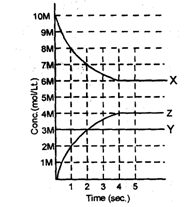Q. Which of the following equilibrium reaction represents the correct variation of concentration with time.

Detailed Solution for Chemical Equation MCQ - 1 (Advanced) - Question 1

Clearly concentration of Y is not changing with the time hence it will be pure solid or liquid. Concentration of X decreasing hence it will be in reactant and Z will be in product.

Chemical Equation MCQ - 1 (Advanced) - Question 2

### X,Y and Z react in the 1 : 1 : 1 stoichiometric ratio. The concentration of X, Y  and Z where found to vary with time as shown in the figure belowQ. Value of the equilibrium constant (Kc) for the equilibrium represented in above sketch will be.

Detailed Solution for Chemical Equation MCQ - 1 (Advanced) - Question 2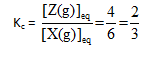Chemical Equation MCQ - 1 (Advanced) - Question 3

### X,Y and Z react in the 1 : 1 : 1 stoichiometric ratio. The concentration of X, Y  and Z where found to vary with time as shown in the figure belowQ. If above equilibrium is established in a 2L container by taking reactants in sufficient amount then how many moles of component Y must have reacted to establish the equilibrium.

Detailed Solution for Chemical Equation MCQ - 1 (Advanced) - Question 3

From the graph we can see that 4 mole/litre of Z is formed therefore      4 × 2 moles of solid will be required.

Chemical Equation MCQ - 1 (Advanced) - Question 4

For certain substances such as ammonium chloride, nitrogen peroxide, phosphorus               pentachloride, etc. the measured densities are found to be less than those calculated from their molecular formula. The observed densities decrease towards a limit as the temperature is raised. This is due to the splitting of the molecules into simpler ones. The process is reversible and is called thermal dissociation.

Examples :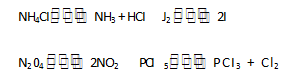With increase in the number of molecules, the volume increases (pressure  remaining constant) and, in consequence, the density decreases. As the temperature rises, more and more dissociation takes place, and when practically complete dissociation occursthe density reaches its lowest limit.The extent of issociation, ice., the fraction of the total number of molecules which suffers dissociation is called the degree of dissociation. Gas density measurements can be used to determine the degree of dissociation. Let us take by general case where one molecule of a substance A splits up into n molecule of B on heating; i.e.,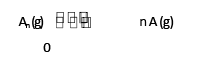t = 0 a

t = teq       a  — x               nx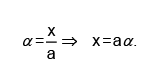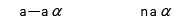Total no. of moles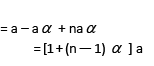Observed molecular weight or molar mass of the mixture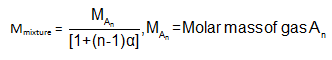Q.

A sample of mixture of A(g), B(g) and C(g) under equilibrium has a mean molecular weight (observed) is 80.

The equilibrium is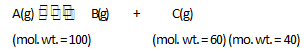Find the degree of dissociation α for A(g).

Detailed Solution for Chemical Equation MCQ - 1 (Advanced) - Question 4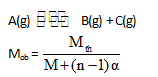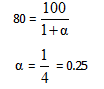Chemical Equation MCQ - 1 (Advanced) - Question 5

For certain substances such as ammonium chloride, nitrogen peroxide, phosphorus               pentachloride, etc. the measured densities are found to be less than those calculated from their molecular formula. The observed densities decrease towards a limit as the temperature is raised. This is due to the splitting of the molecules into simpler ones. The process is reversible and is called thermal dissociation.

Examples :With increase in the number of molecules, the volume increases (pressure  remaining constant) and, in consequence, the density decreases. As the temperature rises, more and more dissociation takes place, and when practically complete dissociation occursthe density reaches its lowest limit.The extent of issociation, ice., the fraction of the total number of molecules which suffers dissociation is called the degree of dissociation. Gas density measurements can be used to determine the degree of dissociation. Let us take by general case where one molecule of a substance A splits up into n molecule of B on heating; i.e.,t = 0 a

t = teq       a  — x               nxTotal no. of molesObserved molecular weight or molar mass of the mixtureQ.

If the total mass of the mixture in the above case is 300 gm, the moles at C(g) present are.

Detailed Solution for Chemical Equation MCQ - 1 (Advanced) - Question 5

Mols of C = a α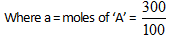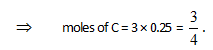Chemical Equation MCQ - 1 (Advanced) - Question 6

For certain substances such as ammonium chloride, nitrogen peroxide, phosphorus               pentachloride, etc. the measured densities are found to be less than those calculated from their molecular formula. The observed densities decrease towards a limit as the temperature is raised. This is due to the splitting of the molecules into simpler ones. The process is reversible and is called thermal dissociation.

Examples :With increase in the number of molecules, the volume increases (pressure  remaining constant) and, in consequence, the density decreases. As the temperature rises, more and more dissociation takes place, and when practically complete dissociation occursthe density reaches its lowest limit.The extent of issociation, ice., the fraction of the total number of molecules which suffers dissociation is called the degree of dissociation. Gas density measurements can be used to determine the degree of dissociation. Let us take by general case where one molecule of a substance A splits up into n molecule of B on heating; i.e.,t = 0 a

t = teq       a  — x               nxTotal no. of molesObserved molecular weight or molar mass of the mixtureQ.

The K for the reaction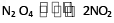is 640 mm at 775 K. The percentage dissociation of N2O4 at equilibrium pressure of 160 mm is :

Detailed Solution for Chemical Equation MCQ - 1 (Advanced) - Question 6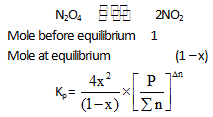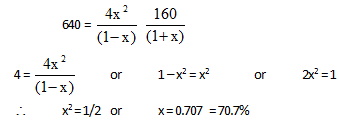Chemical Equation MCQ - 1 (Advanced) - Question 7

For certain substances such as ammonium chloride, nitrogen peroxide, phosphorus               pentachloride, etc. the measured densities are found to be less than those calculated from their molecular formula. The observed densities decrease towards a limit as the temperature is raised. This is due to the splitting of the molecules into simpler ones. The process is reversible and is called thermal dissociation.

Examples :With increase in the number of molecules, the volume increases (pressure  remaining constant) and, in consequence, the density decreases. As the temperature rises, more and more dissociation takes place, and when practically complete dissociation occursthe density reaches its lowest limit.The extent of issociation, ice., the fraction of the total number of molecules which suffers dissociation is called the degree of dissociation. Gas density measurements can be used to determine the degree of dissociation. Let us take by general case where one molecule of a substance A splits up into n molecule of B on heating; i.e.,t = 0 a

t = teq       a  — x               nxTotal no. of molesObserved molecular weight or molar mass of the mixtureQ.

x (degree of dissociation) varies with D/d in the above reaction according to :

Detailed Solution for Chemical Equation MCQ - 1 (Advanced) - Question 7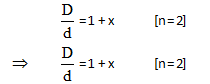Chemical Equation MCQ - 1 (Advanced) - Question 8

For certain substances such as ammonium chloride, nitrogen peroxide, phosphorus               pentachloride, etc. the measured densities are found to be less than those calculated from their molecular formula. The observed densities decrease towards a limit as the temperature is raised. This is due to the splitting of the molecules into simpler ones. The process is reversible and is called thermal dissociation.

Examples :With increase in the number of molecules, the volume increases (pressure  remaining constant) and, in consequence, the density decreases. As the temperature rises, more and more dissociation takes place, and when practically complete dissociation occursthe density reaches its lowest limit.The extent of issociation, ice., the fraction of the total number of molecules which suffers dissociation is called the degree of dissociation. Gas density measurements can be used to determine the degree of dissociation. Let us take by general case where one molecule of a substance A splits up into n molecule of B on heating; i.e.,t = 0 a

t = teq       a  — x               nxTotal no. of molesObserved molecular weight or molar mass of the mixtureQ.

The equation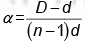is correctly matched for :

Detailed Solution for Chemical Equation MCQ - 1 (Advanced) - Question 8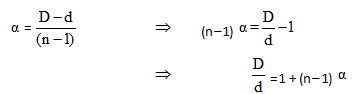Hence one mole of reactant should produce total n moles of product.

Chemical Equation MCQ - 1 (Advanced) - Question 9

Match the following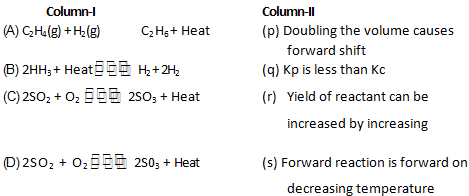Chemical Equation MCQ - 1 (Advanced) - Question 10

Match the following (multiple)

Left column : Represents an equilibrium situratton through a chemical equation and below each equation a stimulus is given which may or may not

disturb the equilibrium situration.

Right coloumn : Represents the responses immediately after the    disturbance is created.

With                                                                      R1 : Rate of forward reaction.

Rb Rate of backward reaction.

Q : Reaction quotient

K : Equilibrium constant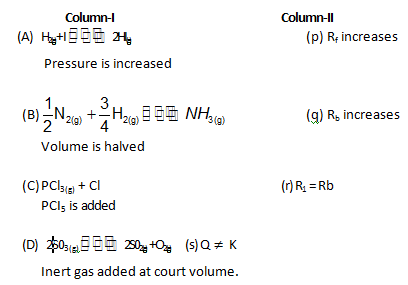## Chemistry for JEE Advanced

12 videos|53 docs|118 tests
 Use Code STAYHOME200 and get INR 200 additional OFF Use Coupon Code
Information about Chemical Equation MCQ - 1 (Advanced) Page
In this test you can find the Exam questions for Chemical Equation MCQ - 1 (Advanced) solved & explained in the simplest way possible. Besides giving Questions and answers for Chemical Equation MCQ - 1 (Advanced), EduRev gives you an ample number of Online tests for practice

## Chemistry for JEE Advanced

12 videos|53 docs|118 tests